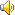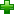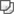# equate [i'kweit]vt. 使相等；视为平等
vi. 等同

# 英英解释

v.
1. consider or describe as similar, equal, or analogous
2. be equivalent or parallel, in mathematics
3. make equal, uniform, corresponding, or matching

# 网络释义

Equate
相等; 使相等; 伊奎特; 使...相等;
equate with
把…等同; 等同; 使相等;
equate to
等量齐观; 折合; 认为相像;
be equal equate
等同;